Physics - Chapter 6 Lesson 46: Momentum and Impulse Notes

LEARNING TARGET JOURNAL

 What are Fitch Barrels? What are they used for?Why should you wear a  helmet?

NOTES

 Momentum is the product of an object’s mass and its velocity.Since velocity is a vector quantity, so then is momentum.                                        p = mvThe direction of the momentum is the same as the direction of the velocity.The variable for momentum is p.                                        The SI units on momentum areAlternate units for momentum areThe change in momentum of an object by applying a force for a given amount of time is called impulse.The variable for impulse is Δp.                                𝛥p = F·𝛥tThe SI units for impulse areWhich, remember, are equivalent to the units for momentum,.

EXAMPLE 1 – Solve the following using momentum.

 A 2250 kg pickup truck has a velocity of 25 m/s to the east. What is the momentum of the truck? What velocity must a car with a mass of 1210 kg have in order to have the same momentum as the pickup truck in Problem 1 above?

EXAMPLE 2 – Solve the following impulse problems.

 A 0.40 kg soccer ball approaches a player horizontally with a velocity of 18 m/s to the north. The player strikes the ball and causes it to move in the opposite direction with a velocity of 22 m/s. What impulse was delivered to the ball by the player? A 0.50 kg football is thrown with a velocity of 15 m/s to the right. A stationary receiver catches the ball and brings it to rest in 0.020 s. What is the force exerted on the receiver?

HOMEWORK -

 Physics - Chapter 6 Lesson 47: Impulse-Momentum Theorem Notes

LEARNING TARGET JOURNAL

 Describe the relationship between force exerted and time of exertion as it applies to impulse and the magnitude of the change in momentum.

NOTES

 Impulse can determine how long a force must be applied to change the velocity of an object.The formula for impulse is more commonly used as:F·𝛥t = mvf - mviLeave space for proof.This can be used in conjunction with the kinematic equations and the Work-Kinetic Energy Theorem to find the displacementand accelerationof the object.

EXAMPLE 1 – Solve for the following using the Impulse-Momentum Theorem.

 A football punter accelerates a 0.55 kg football from rest to a speed of 8.0 m/s in 0.25 s. What constant force does the punter exert on the ball? A 0.15 kg baseball is thrown with a speed of 20 m/s. It is hit straight back at the pitcher with a final speed of 22 m/s. Find the average force exerted by the bat on the ball if the two are in contact for 0.0020 s.

EXAMPLE 2 – A 0.15 kg baseball moving at +26 m/s is slowed to a stop by a catcher who by a constant force of −390 N.

 How long does it take this force to stop the ball? How far does the ball travel before stopping?

HOMEWORK -

 Physics - Chapter 6 Lesson 48: Conservation of Momentum in Collisions Notes

NOTES

 Momentum is the best concept to explain the interaction of objects using Newton’s Laws of Motion.The Law of Conservation of Momentum explains Newton’s 3rd Law of Motion The Law of Conservation of Momentum states that the sum of the momentum of all objects before the collision must be equal to the sum of the momentum of all objects after.A collision is a term to describe any interaction between two or more objects.There are two basic types of collisions:ElasticInelasticAn elastic collision is observed as two objects that collide and both change velocity while neither changes shape.The direction of each object may or may not change.In all elastic collisions, the total kinetic energy of the system must be conserved.To verify a collision is elastic, you verify that the sum of the initial kinetic energies of all objects is equal to the sum of the final kinetic energies.

EXAMPLE 1 – Solve the following using the Law of Conservation of Momentum.

 A 0.015 kg marble moving to the right at 0.225 m/s makes an elastic head-on collision with a 0.030 kg shooter marble moving to the left at 0.180 m/s. After the collision, the smaller marble moves to the left at 0.315 m/s. Assume that neither marble rotates before or after the collision and that both marbles are moving on a frictionless surface. What is the velocity of the 0.030 kg marble after the collision? High-speed stroboscopic photographs show the head of a 215 gram golf club traveling at 55.0 m/s just before it strikes a 46 gram golf ball at rest on a tee. After the collision, the club travels (in the same direction) at 42.0 m/s. Find the speed of the golf ball just after impact.

EXAMPLE 2 – A 16.0 kg canoe moving to the left at 12 m/s makes an elastic head-on collision with a 4.0 kg raft moving to the right at 6.0 m/s. After the collision, the raft moves to the left at 22.7 m/s.

 Find the velocity of the canoe after the collision. Verify your answer by calculating the total kinetic energy before and after the collision.

EXAMPLE 3 - Two 0.40 kg soccer balls collide elastically in a head-on collision. The first ball starts at rest, and the second ball has a speed of 3.5 m/s. After the collision, the second ball is at rest.

 What is the final speed of the first ball?

HOMEWORK -

 Physics - Chapter 6 Lesson 49: Inelastic and Perfectly Inelastic Collisions Notes

LEARNING TARGET JOURNAL

 After observing the cool effects of Newton’s Cradle, answer the following:When one ball is dropped, what happens to the momentum of that ball?When two balls are dropped, how many are kicked out? Why?When 3 balls are dropped, why does the middle ball continue to move?

NOTES

 An inelastic collision is one in which two objects collide with one another and lose a portion of their kinetic energy due to non-restoring forces.This would mean that one or both of the objects will deform or alter their shape during the collision.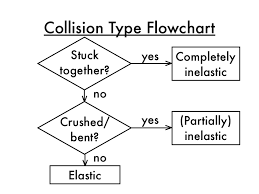We will examine two levels of inelastic collisions.An inelastic collision in which one or both of the objects involved deform during the collision, but travel as two separate masses after the collision.A perfectly inelastic collision is one in which two objects collide and join together to travel at one common velocity.This is best illustrated by catching a baseball or possibly a tackle in football.To verify a collision is inelastic, show that KEi ≠ KEf.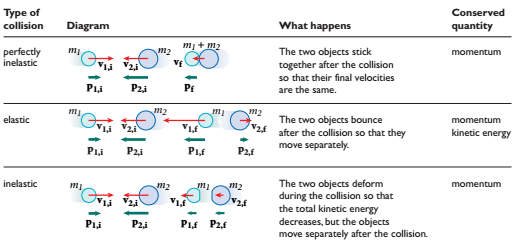EXAMPLE 1 – After being struck by a bowling ball, a 1.5 kg bowling pin sliding to the right at 3.0 m/s collides head-on with another 1.5 kg bowling pin initially at rest.

 Find the final velocity of the second pin when the first pin moves to the right after the collision at 0.5 m/s Calculate the decrease in total kinetic energy as a result of the collision.

EXAMPLE 2 – Solve for the indicated value in the perfectly inelastic collisions.

 An 85.0 kg fisherman jumps from a dock into a 135.0 kg rowboat at rest on the west side of the dock. If the velocity of the fisherman is 4.30 m/s to the west as he leaves the dock, what is the final velocity of the fisherman and the boat? A 90.0 kg fullback moving south with a speed of 5.0 m/s has a perfectly inelastic collision with a 95.0 kg opponent running north at 3.0 m/s. Calculate the velocity of the players just after the tackle. A dry cleaner throws a 22 kg bag of laundry onto a stationary 9.0 kg cart. The cart and laundry bag begin moving at 3.0 m/s to the right. Find the velocity of the laundry bag before the collision.

HOMEWORK -

 Physics - Chapter 6 Lesson 50: Explosions Notes

LEARNING TARGET JOURNAL

 Identify which of Newton’s Three Laws of Motion explains why a cannon moves backwards as it fires.Why does the cannonball go farther than the cannon?

NOTES

 An explosion follows the same principles as a perfectly inelastic collision, only in reverse.For many cases, the initial momentum of the system will be zero before the trigger is pulled or the object is fired because.In this case, the two velocities must be in opposite directions but not necessarily same magnitudes.There are times where an object is launched or thrown from a moving object, and you are asked to find the final speed of either object.In this case, the two velocities could still be in opposite directions or the same direction.

EXAMPLE 1 – Use the Law of Conservation of Momentum in the explosions below.

 A tennis player places a 55 kg ball machine on a frictionless surface, as in the figure at right. The machine fires a 0.057 kg tennis ball horizontally with a velocity of 36 m/s toward the north. What is the final velocity of the machine?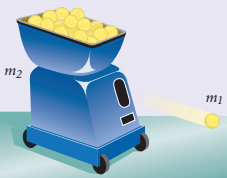A boy on a 2.0 kg skateboard initially at rest tosses an 8.0 kg jug of water in the forward direction. If the jug has a speed of 3.0 m/s relative to the ground and the boy and skateboard move in the opposite direction at 0.60 m/s, find the boy’s mass.

EXAMPLE 2 - A 65.0 kg ice skater moving to the right with a velocity of 2.50 m/s throws a 0.150 kg snowball to the right with a velocity of 32.0 m/s relative to the ground.

 What is the velocity of the ice skater after throwing the snowball?

HOMEWORK -

 Physics - Chapter 6 Lesson 51: Perfectly Elastic Collisions Notes

LEARNING TARGET JOURNAL

 Why can everyday collisions never be truly elastic?Where might there be collisions that are truly elastic?

NOTES

 Remember, an elastic collision can be conserved usingA perfectly elastic collision is when two objects of the same mass collide with one another.The end result is that the two objects will switch roles by switching velocities.Quite often, elastic collision problems ask for the final velocity of both objects after the collision. To conserve the kinetic energy in this case, use the conservation equation from above and the one below in a system of equations to solve for the unknown velocities.EXAMPLE 1 – Two shuffleboard disks of equal mass, one orange and the other yellow, are involved in an elastic collision. The yellow disk is initially at rest and is struck by the orange disk moving initially to the right at 5.00 m/s. After the collision, the orange disk is at rest.

 What is the velocity of the yellow disk after the collision?

EXAMPLE 2 – A 2.0 gram particle moving at 8.0 m/s makes an elastic head-on collision with a resting 1.0 gram particle.

 Find the speed of each particle after the collision.

HOMEWORK -

 Physics - Chapter 6 Lesson 52: Force vs Time Graphs Notes

NOTES

 A Force vs Time Graph measures a varying force at any given time during an event or collision.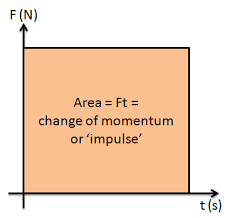The area under the graph represents the change in momentum, or also known as the impulse, on the object.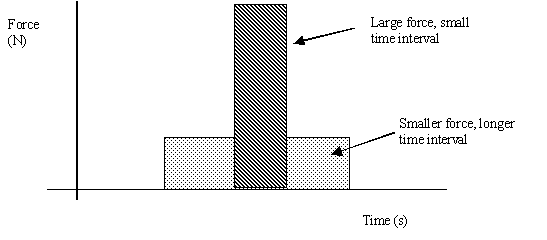The slope of this graph does not give us a Physics quantity of interest.

EXAMPLE 1 – Use the Force vs Time Graph at right to answer the following.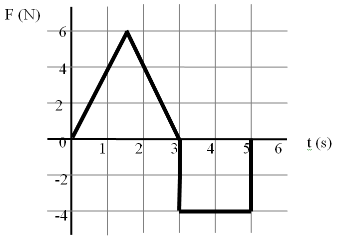Find the impulse imparted on the object during the first 3 seconds. Find the impulse imparted on the object during the last 2 seconds. Calculate the overall change in momentum of the object. Describe a scenario that would create a graph like this.

HOMEWORK -